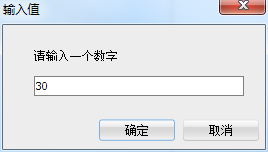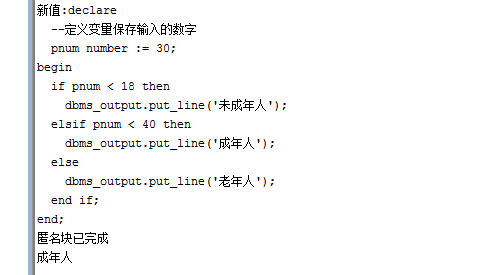PL/SQL  IF條件判斷語句

1、IF 語法：

IF 條件 THEN 語句;
END IF;

--開啟螢幕輸出開關（預設沒有開啟的）
set serveroutput on;
--接受鍵盤輸入，變數num：是一個地址值，在該地址上儲存了輸入的值
accept num prompt '請輸入一個數字';

declare
--定義變數儲存輸入的數字
pnum number := &num;
begin
if pnum = 1 then
dbms_output.put_line('我是數字1');
end if;
end;
/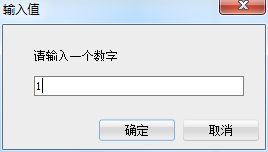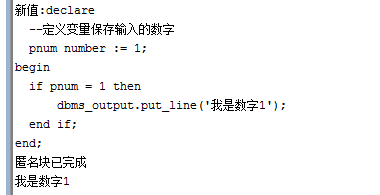2、IF ... ELSE 語法：

IF 條件 THEN 語句;
ESLE 語句;
END IF;

--開啟螢幕輸出開關（預設沒有開啟的）
set serveroutput on;
--接受鍵盤輸入，變數num：是一個地址值，在該地址上儲存了輸入的值
accept num prompt '請輸入一個數字';

declare
--定義變數儲存輸入的數字
pnum number := &num;
begin
if pnum = 1 then
dbms_output.put_line('我是數字1');
else
dbms_output.put_line('我不是數字1');
end if;
end;
/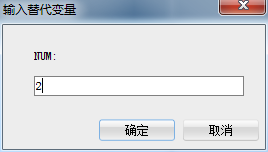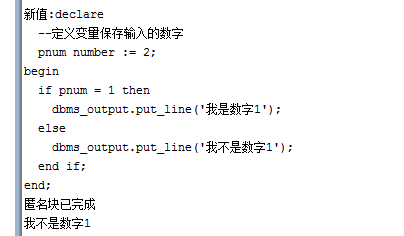3、IF ... ELSIF ... ELSE 語法：

IF 條件 THEN 語句;
ELSIF 條件 THEN 語句;
ELSE 語句;
END IF;

--開啟螢幕輸出開關（預設沒有開啟的）
set serveroutput on;
--接受鍵盤輸入，變數num：是一個地址值，在該地址上儲存了輸入的值
accept num prompt '請輸入一個數字';

declare
--定義變數儲存輸入的數字
pnum number := &num;
begin
if pnum < 18 then
dbms_output.put_line('未成年人');
elsif pnum < 40 then
dbms_output.put_line('成年人');
else
dbms_output.put_line('老年人');
end if;
end;
/